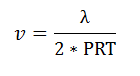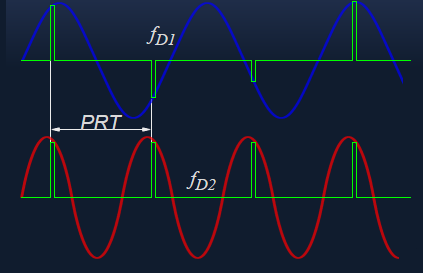This CalcTown calculator calculates the radar blind speed according to the blind speed equation.

If the Doppler frequency produced by a moving target is exactly the same as PRF, then sampling occurs at the same point in each cycle. The blind speed is a radial speed of the airplane at which the phase shifting of the echo-signal has the value ±n · 360° between two pulse periods. With blind speeds moving targets are suppressed by a MTI system like ground clutters.

#### Result

m/sWhere,

f = frequecy of operation

PRT = pulse repetition time

Due to the periodicity of a measured Doppler frequency is only in the period from zero to the first maximum of the sine wave (representing the Doppler frequency) unambiguously assigned to a velocity. The Doppler frequency must be lower than the pulse repetition frequency. This ambiguity in measurements of velocity is called Doppler Dilemma.

Simple measures against the appearance of blind speeds are:

Using of the coherence channel only if it is necessary

This demand also arises that the amplitude channel („Normal Video”) has a better maximum range than the „Coho-channel”.

Constantly changing of the TX- frequency (Frequency-Diversity)

It is demanded a sufficiently large frequency spacing.

Constantly changing of the pulse repetition time (Staggered PRT)

Most effective resource: no airplane can change its speed so fast and synchronous.